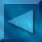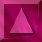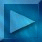Previous : The MCMCML estimation algorithm  Next : Estimation results Notations

 The ICM-DCT reconstruction algorithm

 This deterministic algorithm is based on the posterior modified Geman & Yang sampler,  whose random part is suppressed.

We use the same half-quadratic form of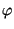: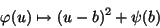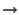auxiliary variable b, auxiliary images Bx and By (gradients w.r.t. x and y)alternated minimization w.r.t. X and Bx, By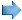The criterion to optimize can be rewritten as follows :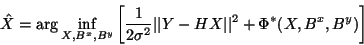with the new regularization term :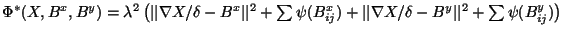The ICM-DCT reconstruction algorithm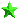Initialization :  Let us first calculate the Fourier transform F[h4 ] and DCT[Y], and W :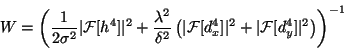The initialization is done using X0 = Y  (observed image).Minimization of the criterion w.r.t. Bx,By : The pixels b of Bx and By are independent :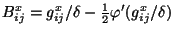et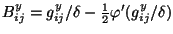Minimisation of the criterion w.r.t. X : The criterion is a quadratic form w.r.t. X :diagonalization by a cosine transform (DCT)           which enables to fulfill symmetric boundary conditions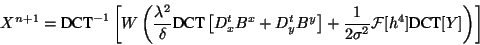Example (64x64 image) :

 X0initialization  X0 = Y XB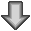Bx 0, By 0BX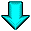iteration 1 X1XBBx 1, By 1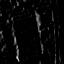BXiteration 2 X2etc. N iterations before convergence usually N<10

 Example :  64x64 synthetic image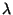= 0.25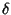= 5 Example : 64x64 image= 0.45= 10012345678910012345678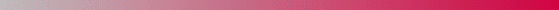André Jalobeanu - 24 Aug 1998